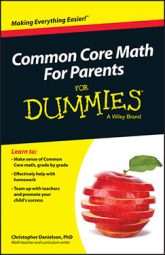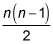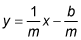##### Common Core Math For Parents For Dummies with Videos OnlineConnecting ideas is an important part of the Common Core Standards for Mathematics. This is because math is rich with connections. Connections basically are relationships between ideas. But math is often taught in a way that obscures these connections.

When teachers insist on memorizing both addition and subtraction facts (which is different from insisting that students be able to produce these facts quickly), they obscure the connections between addition and subtraction, for example. If you know that 8 + 4 = 12 and you know the connections between addition and subtraction, then you can quickly produce 4 + 8, 12 – 4, and 12 – 8 because they're all connected examples of one relationship.

In fact, mathematics can connect what seem to be very different situations. When you build a mathematical model of a situation, you strip away details. For example, when you have three red apples and four green apples and you write 3 + 4, you strip away the fact that this is an idea about apples. Then, when you count four spaces to the right on the number line, starting at 3, and you write 3 + 4 again, you have a connection. This relationship — that 3 + 4 = 7 — is true of a wide variety of contexts.

The following two questions are common in algebra and geometry classes:

• If n people are on a basketball team and each one gives the others one high five, how many high fives are given altogether?

• How many diagonals can you draw in a regular polygon with n sides?

In both cases, the answer is the same:After you have enough experience with these types of problems, you can start to have hunches about which kinds of mathematical models are likely to be useful for different situations. Through repeated experiences with modeling, you can get better at noticing the structure of a problem situation.

In addition to thinking of algebra as generalized arithmetic (this means that algebra answers questions about all numbers, not just the numbers in a particular computation), you can think of algebra as an efficient way of getting things done. Algebra can capture the regularity in repeated reasoning. In order to capture it, you need to look for connections.

For example, when students study inverse functions in high school, they can notice the connections between the inverses they find for linear functions. Namely, that the inverse of a linear function y = mx + b is also linear and has a particular form:This observation is motivated by repeatedly reasoning your way to a solution for particular linear functions and eventually asking the questions, "What is the same in each of these problems? How are these connected?"

A simpler example of this Standard for Mathematical Practice about regularity in repeated reasoning occurs when students move from counting to solving problems such as 9 + 2, 9 + 3, and 9 + 4 to having strategies for knowing the sum of 9 and any one‐digit number. Students frequently notice that these sums come out as 10 plus a number one less than the original number. That is 9 + 2 = 11, and 11 is 10 + 1. Before students have memorized all of their single‐digit addition facts, they frequently have noticed the regularity in their repeated reasoning about sums involving 9 — and similar patterns in sums involving other numbers.